×#### Thank you for registering.

One of our academic counsellors will contact you within 1 working day.

Click to Chat

1800-1023-196

+91-120-4616500

CART 0

• 0

MY CART (5)

Use Coupon: CART20 and get 20% off on all online Study Material

ITEM
DETAILS
MRP
DISCOUNT
FINAL PRICE
Total Price: Rs.

There are no items in this cart.
Continue Shopping• Complete JEE Main/Advanced Course and Test Series
• OFFERED PRICE: Rs. 15,900
• View Details

```Chapter 20: Surface Area And Volume of A Right Circular Cone Exercise – 20.2

Question: 1

Find the volume of the right circular cone with the following dimensions:

(a) Radius is 6 cm and the height of the cone is 7cm

(b) Radius is 3.5 cm and height is 12 cm

(c) Slant height is 21 cm and height is 28 cm

Solution:

(a) It is given that

Radius of the cone (r) = 6 cm

Height of the cone (h) = 7 cm

Volume of a right circular cone

= 1/3πr2h

= 1/3 ∗ 3.14 ∗ 62 ∗ 7 = 264 cm3

(b) It is given that:

Radius of the cone (r) = 3.5 cm

Height of the cone (h) = 12 cm

Volume of a right circular cone

= 1/3πr2h

= 1/3 ∗ 3.14 ∗ 3.52 ∗ 12

= 154 cm3

(c) It is given that:

Height of the cone (h) = 28 cm

Slant height of the cone (l) = 21 cm

As we know that,

l2 = r2 + h2Volume of a right circular cone:

= 1/3πr2h= 7546 cm3

Question: 2

Find the volume of a conical tank with the following dimensions in liters:

(a) Radius is 7cm and the slant height of the cone is 25 cm

(b) Slant height is 12cm and height is 13 cm

Solution:

(a) It is given that

Radius of the cone(r) = 7 cm

Slant height of the cone (l) = 25 cm

As we know that,

l2 = r2 + h2= 24 cm

Volume of a right circular cone

= 1/3 πr2h

= 1/3 ∗ 3.14 ∗ 72 ∗ 24

= 1232 cm3 = 1.232 litres [1 cm3 = 0.01l]

(c) It is given that:

Height of the cone (h) = 12 cm

Slant height of the cone (l) = 13 cm

As we know that,

l2 = r2 + h2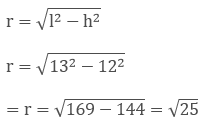= 5 cm

Volume of a right circular cone:

= 1/3 πr2h

= 1/3 ∗ 3.14 ∗ 52 ∗ 10 = 314.85 cm3 = 0.307 litres   [1 cm3 = 0.01l]

Question: 3

Two cones have their heights in the ratio 1:3 and the radii of their bases in the ratio 3:1.Find the ratio of their volumes.

Solution:

Let ratio of the height of the cone be hx

height of the 1st cone = hx

height of the of the  2nd cone =3hx

Let the ratio of the radius of the of the cone = rx

radius of the 1st cone = 3 rx

radius of the 2nd cone = rx

The ratio of the volume = v1/v2

Where v1 = volume of 1st cone

v2 = volume of 2nd cone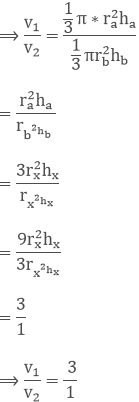Question: 4

The radius and the height of a right circular cone are in the ratio 5:12. If its volume is 314 cubic meter, find the slant height and the radius. (Use π = 3.14).

Solution:

Let us assume the ratio to be y

Height (h) = 12y

We know that

l2 = r2 + h2

= 5y2 + 12y2

= 25y2 + 144y2

= 1692 = 13y

Now it is given that volume = 314 m3

⟹ 1/3πr2h = 314 m3

⟹ 1/3 ∗ 3.14 ∗ 25y2 ∗ 12y = 314 m3

⟹ y3 = 1

⟹ y = 1

Therefore,

Slant height (l) = 13y = 13 m

Radius = 5y = 5 m

Question: 5

The radius and height of a right circular cone are in the ratio 5:12 and its volume is 2512 cubic cm. Find the slant height and radius of the cone. (Use π = 3.14).

Solution:

Let the ratio be y

The radius of the cone(r) = 5y

Height of the cone = 12y

Now we know,= 13y

Now the volume of the cone is given 2512 cm3

⟹ 1/3 πr2h = 2512

⟹ 1/3 ∗ 3.14 ∗ 5y2 ∗ 12y = 2512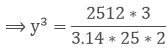⟹ y = 2

Therefore,

Slant height (l) = 13y = 13 * 2 = 26 cm

Radius of cone = 5y = 5 * 2 = 10 cm

Question: 6

The ratio of volumes of two cones is 4:5 and the ratio of the radii of their bases is 2:3. Find the ratio of their vertical heights.

Solution:

Let the ratio of the radius be x

Radius of 1st cone = 2x

Radius of 2nd cone = 3x

Let the ratio of the volume be y

Volume of 1st cone = 4y

Volume of 2nd cone = 5y

y1/y2 = 4y/5y

= 4/5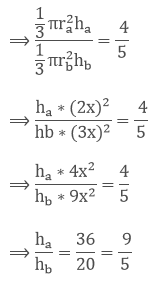Therefore the heights are in the ratio of 9:5.

Question: 7

A cylinder and a cone have equal radii of their bases and equal heights. Show that their volumes are in the ratio 3:1.

Solution:

It's given that

A cylinder and a cone are having equal radii of their bases and heights

Let the radius of the cone =radius of the cylinder = r

Height of the cone=height of the cylinder = h

Let the volume of cone = vx

Volume of cylinder = vy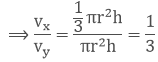⟹ vy/vx = 3/1

Therefore the ratio of their volumes is 3:1.

Question: 8

If the radius of the base of a cone is halved, keeping the height same, what is the ratio of the volume of the reduced cone to that of the original cone?

Solution:

Let the radius of the cone be rx and height be hx

Then the volume of cone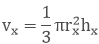Now,

Radius of the reduced cone = rx/2

Therefore volume of reduced cone vy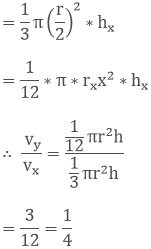Therefore the ratio between the volumes of the reduced and the original cone is 1: 4.

Question: 9

A heap of wheat is in the form of a cone of diameter 9 m and height 3.5 m. Find its volume. How much is canvas cloth required to just cover the heap?  (Use π = 3.14).

Solution:

It is given that

Diameter of heap (d) = 9 m

Therefore, Radius of the heap (r)

= d/2

= 9/2 = 4.5 m

Height of the heap (h) = 3.5 m

Therefore, Volume of the heap = 1/3πr2h

= 1/3 ∗ 3.14 ∗ 4.52 ∗ 3.5

= 74.18 m3

Now,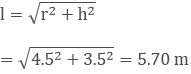Area to be covered by the cloth = Curved surface area of the heap

= πrl = 3.14 * 4.5 * 5.70 = 80.54 m3

Question: 10

Find the weight of a solid cone whose base is of diameter 14 cm and vertical height 51 cm, supposing the material of which it is made weighs 10 grams per cubic cm.

Solution:

It is given that:

Diameter (d) = 14 cm

Height of the cone (h) = 51cm

Radius of the cone(r) = d/2

= 14/2 = 7cm

Therefore, Volume of cone (v) = 1/3πr2h

= 1/3 * 3.14 * 7 * 5 * 51 = 2618 cm3

Now it is given that 1 cm3 material weighs 10 gm.

Therefore, 2618 cm3 weighs = 2618 * 10 = 26180 grams.

Question: 11

A right angled triangle of which the sides containing the right angle are 6.3 cm and 10 cm in length, is made to turn round on the longer side. Find the volume of the solid, thus generated. Also, find its curved surface area.

Solution:

It is given that

Radius of cone(r) = 6.3 cm

Height of the cone (h) = 10 cm

We know that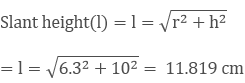Therefore Volume of cone (v) = 1/3πr2h

= 1/3 ∗ 3.14 ∗ 6.32 ∗ 10 = 415.8 cm3

Curved surface area of cone = πrl

= 3.14 * 6.3 * 11.819 = 234.01cm2

Question: 12

Find the volume of the largest right circular cone that can be fitted in a cube whose edge is 14 cm.

Solution:

Radius of the base of the largest cone = 1/2 * edge of the cube

= 1/2 *14 = 7 cm

Height of the cone = Edge of the cube = 14 cm

Therefore, Volume of cone (v) = 1/3πr2h

= 1/3 ∗ 3.14 ∗ 72 ∗ 14 = 718.66 cm3

Question: 13

The volume of a right circular cone is 9856 cm3.If the diameter of the base is 28 cm. Find:

(a) Height of the cone

(b) Slant height of the cone

(c) Curved surface area of the cone

Solution:

(a) It is given that diameter of the cone (d) = 28 cm

Radius of the cone(r) = d/2

= 28/2 = 14cm

Height of the cone = ?

Now,

Volume of the cone (v) = 1/3πr2h = 9856 cm3

⟹ 1/3 ∗ 3.14 ∗ 142 ∗ h = 9856Therefore the height of the cone is 48 cm

(b) It is given that

Radius of the cone(r) = 14 cm

Height of the cone = 48 cm

Slant height (l) = ?

Now we know thatTherefore the slant height of the cone is 50 cm.

(c) Radius of the cone(r) = 14 cm

Slant height of the cone (l) = 50 cm

Curved surface area (C.S.A) = ?

Curved surface area of a cone (C.S.A) = πrl

= 3.14 * 14 * 50 = 2200 cm2

Therefore curved surface of the cone is 2200 cm2

Question: 14

A conical pit of top diameter 3.5 m is 12 m deep. What is its capacity in kilolitres?

Solution:

It is given that:

Diameter of the conical pit (d) = 3.5 m

Height of the conical pit (h) = 12 m

Radius of the conical pit (r) = ?

Volume of the conical pit (v) = ?

Radius of the conical pit(r) = d/2 = 3.5/2 = 1.75 m

Volume of the cone (v) = 1/3πr2h

= 1/3 ∗ 3.14 ∗ 1.752 ∗ 12 = 38.5 m3

Capacity of the pit = (38.5 * 1) kilolitres = 38.5 kilolitres

Question: 15

Monica has a piece of Canvas whose area is 551m2. She uses it to have a conical tent made, with a base radius of 7m. Assuming that all the stitching margins and wastage incurred while cutting amounts to approximately 1 m2. Find the volume of the tent that can be made with it.

Solution:

It is given that:

Area of the canvas = 551m2

Area that is wasted = 1m2

Radius of tent = 7m, Volume of tent (v) = ?

Therefore the Area of available for making the tent = (551 - 1) = 550 m2

Surface area of tent = 550 m2

⟹ πrl = 550

⟹ l = 550/22 = 25 m

Slant height (l) = 25 m

We know that,

l2 = r2 + h2

252 = 72 + h2

⟹ 625 - 49 = h2

⟹ 576 = h2

h = 24 m

Height of the tent is 24 m.

Now, volume of cone = 1/3πr2h

= 1/3 ∗ 3.14 ∗ 72 ∗ 24 = 1232 m3

Therefore the volume of the conical tent is 1232 m3.
```### Course Features

• 728 Video Lectures
• Revision Notes
• Previous Year Papers
• Mind Map
• Study Planner
• NCERT Solutions
• Discussion Forum
• Test paper with Video Solution